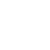# PIC12F1822 DAC module example with CCS C compiler

This small post shows how to start using PIC12F1822 DAC (Digital-to Analog Converter) module.
PIC12F1822 microcontroller has 1 DAC module. The DAC can be used to supply analog voltage on RA0 pin with 32 selectable output levels.

The input of the DAC can be connected to:

• External VREF pins
• VDD supply voltage
• FVR (Fixed Voltage Reference)

DAC Block diagram is shown below:

With CCS PIC C compiler we can initialize the DAC module using the following command:
setup_dac(int8 mode);
Where mode can one of the following modes:
DAC_OFF  0                  // DAC disabled
DAC_VSS_VDD               // Negative source is VSS and positive source is VDD
DAC_VSS_VREF              // Negative source is VSS and positive source is Vref+ pin
DAC_VSS_FVR               // Negative source is VSS and positive source is FVR (Fixed Voltage Reference)

The DAC output can be enabled using the following line which or’ed with above in setup_dac() using |.
DAC_OUTPUT               // Enable ADC output on RA0 pin.
Controlling the output of the DAC is also easy with CCS PIC C compiler and for that we have to use the following command:
dac_write(int8 value);
Where value should be a 5-bit number which varies between 0 and 31.
The DAC output voltage is determined by the following equation assuming that the DAC is enabled (DACEN bit is 1):

The DAC 32 levels are set with DACR[4:0] bits which is done using dac_write() command.
Assume that we’ve PIC12F1822 microcontroller with +5V power supply, the DAC is configured with VSS and VDD as negative and positive sources and the DAC output is enabled.
Minimum Vout voltage when DACR[4 : 0] = 0b00000 equals to 0V.
Maximum Vout voltage when DACR[4 : 0] = 0b11111 equals to 4.84V
Writing DACR[4 : 0] = 0b00000 is done using: dac_write(0);
And DACR[4 : 0] = 0b11111 is dac_write(31);

PIC12F1822 DAC Module Example:
This is a small example for the DAC module. Example circuit schematic is shown below.PIC12F1822 internal oscillator is used.
A potentiometer is used to control the DAC output.
A voltmeter is connected between pin RA0 which is the DAC output (DACOUT) and the ground to see the variation of the voltage according to the potentiometer position.

PIC12F1822 DAC Module Example CCS C Code:

PIC12F1822 DAC module example Proteus simulation video:
The following video shows simulation of our example with Proteus.

### 2 thoughts on “PIC12F1822 DAC module example with CCS C compiler”

1.Pedro Galvez

Hi! I think that in your code the DAC should be set as DAC_VSS_VDD
Great Post!!!

1.Simple Projects

Corrected, thanks.

This site uses Akismet to reduce spam. Learn how your comment data is processed.

Scroll to Top